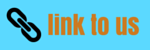# Center of mass equation and Motion of Center of mass

## (4) center of mass equation (Position vector of center of mass in terms of co-ordinate components)

• Let in a system of many particles the co-ordinates of center of mass of the system be (Xcm,Ycm,Zcm) then position vector of centre of mass would be
Rcm=Xcmi+Ycmj+Zcmk                    (9)
and position vectors of various particles would be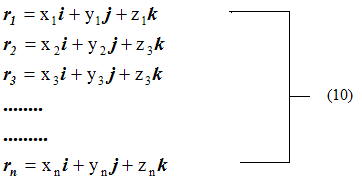• Putting the values from equation 9 and 10 in equation 6, 7 and 8 we get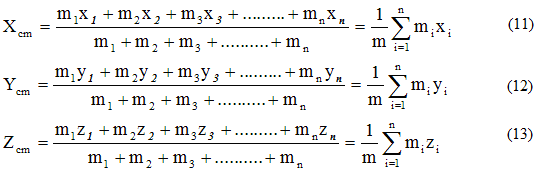• If in any system there are infinite particles of point mass and are distributed continuously also if the distance between them is infinitesimally small then summation in equations 6, 11, 12 and 13 can be replaced by integration. If r is the position vector of very small particle of mass dm of the system then position vector of centre of the system would be
and the value of its co-ordinates would be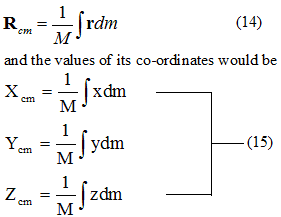• If ρ is the density of the system then dm=ρdV where dV is the very small volume element of the system then,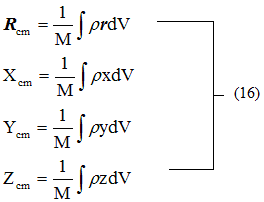• The center of mass of a homogeneous body (body having uniform distribution of mass) must coincide with the geometrical center of the body. In other words we can say that if the homogeneous body has a point , a line or plane of symmetry , then its centre of mass must lie at this point , line or plane of symmetry.
• The center of mass of irregular bodies and shape can be found using equations 14, 15, 16.

## (5) Motion of center of mass

• Differentiating equation $${\vec{R}_{CM}} = \frac{{\sum\limits_{i = 1}^n {{m_i}{{\vec r}_i}} }}{{\sum\limits_{i = 1}^n {{m_i}} }}$$ we get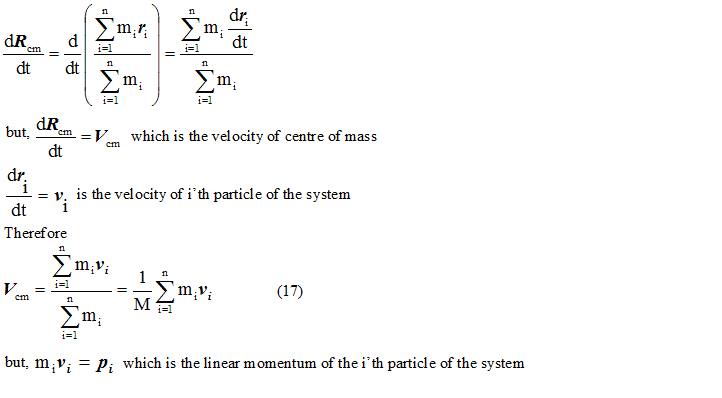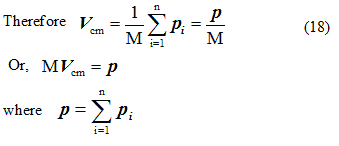p is the vector sum of linear momentum of various particles of the system or it is the total linear momentum of the system.
• If no external force is acting on the system then its linear momentum remains constant. Hence in absence of external force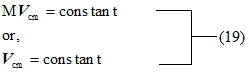• In the absence of external force velocity of centre of mass of the system remains constant or we can say that centre of mass moves with the constant velocity in absence of external force.
• Hence from equation 18 we came to know that the total linear momentum of the system is equal to the product of the total mass of the system and the velocity of the centre of mass of the system which remains constant.
• Thus in the absence of the external force it is not necessary that momentum of individual particles of system like p1 , p2 , p3 . . . . . . . . . . . pn etc. remains constant but their vector sum always remains constant.

## (6) Acceleration of center of mass

• Differentiating equation 7 we get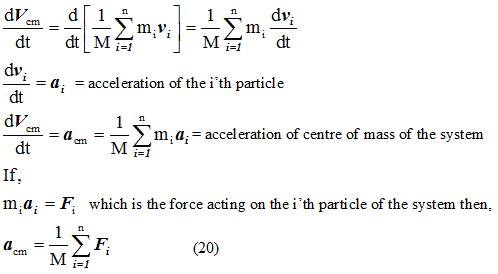• If miai = Fi which is the force acting on the i'th particle of the system then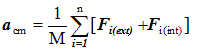• Net force acting on the i'th is
Fi=Fi(ext)+Fi(int)
Here internal force is produced due to the mutual interaction between the particles of the system. Therefore, from Newton's third law of motion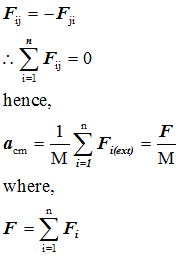is the total external force acting on the system since internal force on the system because of mutual interaction between the particles of the system become equal to zero because of the action reaction law.
• Hence from equation 23 it is clear that the centre of mass of the system of particles moves a if the whole mass of the system were concentrated at it. This result holds whether the system is a rigid body with particles in fixed position or system of particles with internal motions.

Watch this tutorial for information on How to solve Center of Mass problems